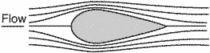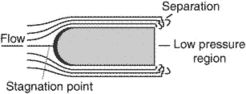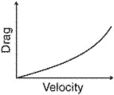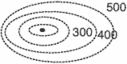# form drag

Also found in: Dictionary, Wikipedia.
Related to form drag: Induced drag, Wave drag

## form drag

[′fȯrm ‚drag]
(fluid mechanics)
The drag from all causes resulting from the particular shape of a body relative to its direction of motion, as of fuselage, wing, or nacelle.
At supersonic speed, the drag caused by losses due to shock waves, exclusive of losses due to skin friction.

## form dragFlow over low drag body.Flow over body with high form drag.Relationship between form drag and speed.Form lines are broken/dotted lines and sketched from visual observations.
A type of parasitic drag. Form drag is caused by the frontal area of the airplane components being exposed to the airstream. Drag is caused by the form or shape of the body and, hence, the name. It can be reduced by streamlining the exposed parts and by area ruling. Also called boundary-layer normal-pressure drag. See also area rule.
References in periodicals archive ?
The form drag force, [F.sub.R], is given by equation (5):
The form drag is a function of the Froude number, ([C.sub.R](Fr)), defined as, equation (8):
The Froude number is used to determine the form drag resistance of ships with different lengths and velocities.
Equation (8) clearly shows that, as the Froude number decreases (i.e., the form drag decreases), the length of a ship increases when the speed is constant.
The relative contribution to the total resistance from the form drag and skin friction of a typical naval ship is shown in Fig.
Figure 3 provides an example of a wake (at stern) and wave (along hull) pattern, which is responsible for the form drag, for a model of the KRISO container ship (KCS) with a Froude number of 0.26.
The form drag and skin friction are the primary resistances responsible for the fuel consumption where only the skin friction is significantly affected by biofouling on the hull surface.
Typically, a flat plate is used when determining skin friction, and a ship model is used when determining the form drag of a specific ship geometry.
where [F.sub.i.sup.f] is the friction drag on (i, i + 1)for sphere i, and [F.sub.i.sup.f] is the form drag on (i, i + 1)for sphere i, [C.sub.f] (15), (16) (34) (35) and Cp  are, respectively, the air friction drag coefficient and form drag coefficient, [p.sub.a] is the air density, [A.sub.n.sup.i] refers to the surface area of the fiber section in the fiber direction, N, is the cross-sectional area of the fiber section, ([u.sub.t]-[v.sub.it]) is the tangential direction relative velocity between the air and sphere, ([u.sub.n]-[v.sub.in]) is the normal direction relative velocity between the air and sphere.
[F.sub.p] form drag force exerted on the fiber section, N

Site: Follow: Share:
Open / Close